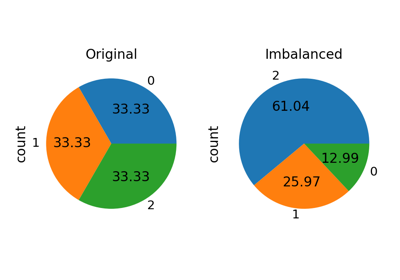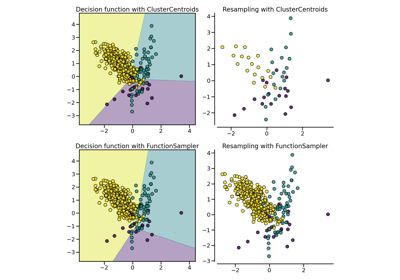# imblearn.under_sampling.RandomUnderSampler¶

class imblearn.under_sampling.RandomUnderSampler(*, sampling_strategy='auto', random_state=None, replacement=False)[source]

Class to perform random under-sampling.

Under-sample the majority class(es) by randomly picking samples with or without replacement.

Read more in the User Guide.

Parameters
sampling_strategyfloat, str, dict, callable, default=’auto’

Sampling information to sample the data set.

• When float, it corresponds to the desired ratio of the number of samples in the minority class over the number of samples in the majority class after resampling. Therefore, the ratio is expressed aswhereis the number of samples in the minority class andis the number of samples in the majority class after resampling.

Warning

float is only available for binary classification. An error is raised for multi-class classification.

• When str, specify the class targeted by the resampling. The number of samples in the different classes will be equalized. Possible choices are:

'majority': resample only the majority class;

'not minority': resample all classes but the minority class;

'not majority': resample all classes but the majority class;

'all': resample all classes;

'auto': equivalent to 'not minority'.

• When dict, the keys correspond to the targeted classes. The values correspond to the desired number of samples for each targeted class.

• When callable, function taking y and returns a dict. The keys correspond to the targeted classes. The values correspond to the desired number of samples for each class.

random_stateint, RandomState instance, default=None

Control the randomization of the algorithm.

• If int, random_state is the seed used by the random number generator;

• If RandomState instance, random_state is the random number generator;

• If None, the random number generator is the RandomState instance used by np.random.

replacementbool, default=False

Whether the sample is with or without replacement.

NearMiss

Undersample using near-miss samples.

Notes

Supports multi-class resampling by sampling each class independently. Supports heterogeneous data as object array containing string and numeric data.

Examples

>>> from collections import Counter
>>> from sklearn.datasets import make_classification
>>> from imblearn.under_sampling import RandomUnderSampler
>>> X, y = make_classification(n_classes=2, class_sep=2,
...  weights=[0.1, 0.9], n_informative=3, n_redundant=1, flip_y=0,
... n_features=20, n_clusters_per_class=1, n_samples=1000, random_state=10)
>>> print('Original dataset shape %s' % Counter(y))
Original dataset shape Counter({1: 900, 0: 100})
>>> rus = RandomUnderSampler(random_state=42)
>>> X_res, y_res = rus.fit_resample(X, y)
>>> print('Resampled dataset shape %s' % Counter(y_res))
Resampled dataset shape Counter({0: 100, 1: 100})

Attributes
sample_indices_ndarray of shape (n_new_samples)

Indices of the samples selected.

New in version 0.4.

__init__(self, *, sampling_strategy='auto', random_state=None, replacement=False)[source]

Initialize self. See help(type(self)) for accurate signature.

## Examples using imblearn.under_sampling.RandomUnderSampler¶